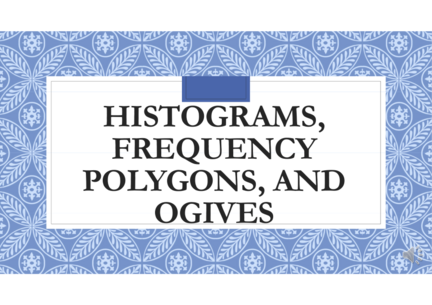# Histograms, Frequency polygons and OgivesContributed by:This pdf includes the following topics:-
Histogram with examples
The frequency polygon with examples
The Ogive
Cumulative Frequency
1. HISTOGRAMS,
FREQUENCY
POLYGONS, AND
OGIVES
2. The histogram is a graph that displays the data by using contiguous vertical
bars (unless the frequency of a class is 0) of various heights to represent the
frequencies of the classes.
Example 1
Construct a histogram to represent the data shown for the record high
temperatures for each of the 50 states
3.
4.
5. 2- The Frequency Polygon
The frequency polygon is a graph that displays the data by using lines that
connect points plotted for the frequencies at the midpoints of the classes.
Example 2
Using the frequency distribution given in Example 1, construct a
frequency polygon.
Step 1 Find the midpoints of each class.
6.
7. Step 4 Connect adjacent points with line segments. Draw a line back to the x
axis at the beginning and end of the graph, at the same distance that the
previous and next midpoints would be located
8. 3- The Ogive
This type of graph is called the cumulative frequency graph, or ogive.
The cumulative frequency is the sum of the frequencies accumulated
up to the upper boundary of a class in the distribution.
The ogive is a graph that represents the cumulative frequencies for the
classes in a frequency distribution.
9. Example 3
Using the frequency distribution given in Example 1, construct an ogive.
10. Step 3 Plot the cumulative frequency at each upper class boundary, as
shown in Figure a. Upper boundaries are used since the cumulative
frequencies represent the number of data values accumulated up to the
upper boundary of each class.
11. Fig. a
12. Step 4 Starting with the first upper class boundary, 104.5, connect adjacent points
with line segments, as shown in Figure b. Then extend the graph to the first lower
class boundary, 99.5, on the x axis.
Fig. b
13. Fig. c